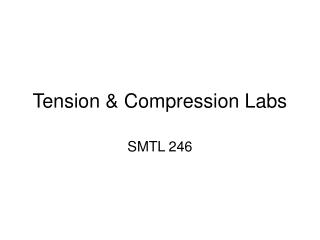DownloadDownload PresentationTension & Compression Labs

# Tension & Compression Labs

Download Presentation## Tension & Compression Labs

- - - - - - - - - - - - - - - - - - - - - - - - - - - E N D - - - - - - - - - - - - - - - - - - - - - - - - - - -
##### Presentation Transcript

1. Tension & Compression Labs SMTL 246

2. These are the results from one of the tension labs

3. These are the results from one of the tension labs The top-left curve is for the mild steel and the bottom-right curve is for the cast iron

4. These are the results from one of the tension labs The top-left curve is for the mild steel and the bottom-right curve is for the cast iron

5. Draw horizontal and vertical axes through the bottom end of the mild steel curve The horizontal axis should also pass through the bottom of the cast iron curve Label the axes

6. Now, we add the units to the axes. The graph paper has mm and cm markings. For the vertical load axis: For the horizontal axis, the scale is 250:1 This means that 250 cm on the paper is equal to 1 cm deformation in the sample … so 1 cm on the paper is equal to 0.004 cm in the sample … or 0.04 mm

7. Place units all the way up the vertical axis and a little way along the horizontal axis. Don’t go as far as the cast-iron curve; we will need to start at 0 again there

8. Draw a line along the proportional part of the curve, approximating the slope as accurately as possible

9. Now, find the slope by measuring the rise (change in load) over the run (change in deformation). Make a large triangle by adding vertical and horizontal lines (a small triangle is less accurate since any error in the reading is magnified).

10. My (rough) measurements from the scan of the graph give me a rise of 15.5 cm ( = 73.2 kN) and a run of 3.35 cm ( = 0.134 mm). We want to calculate E, the modulus of elasticity.

11. To find the yield strength, use the 0.2% offset method (this value is for steel – use 0.05% for cast iron) Draw a line parallel to the proportional part of the curve through the point on the horizontal axis representing 0.2% strain. This line intersects the curve at the yield point. Where is the point that represents 0.2% strain?

12. Now, calculate the stress at the yield point based on the load and the original cross-sectional area

13. Repeat the process for the cast-iron. Reset the origin to the point where the curve crosses the horizontal axis. Do the same process for the compression test. The horizontal scale is 1000:1